Feasibility Study of an LED-Based Lighting System Using Analytical Modeling

By Norman Quesnel - Advanced Thermal Solutions, Inc.

Outdoor LED lighting systems must not only withstand rain and dust, but also solar radiation. In general, LED lighting systems are calculated with no solar load. This is a valid assumption if the lighting systems only operate at night. This article discusses the analytical modeling of an LED-based lighting system for an outdoor application when subjected to various environmental conditions.

Lighting System

The system consists of a rotatable LED-based lamp fixture which is mounted in an enclosure. The enclosure consists of an aluminum compartment on which a dome is placed. The LED lamp fixture is an actively cooled heatsink with 25 cold white LEDs. The junction temperatures of the LEDs need to be determined for different forward currents. Inside the enclosure, components such as motors, drivers and an LED power supply unit (PSU) dissipate heat.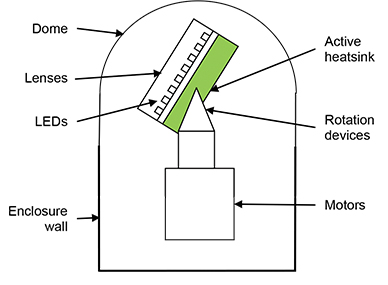Figure 1: Sketch of the lighting system.

Analytical Model

A thermal resistance diagram is derived to analyze the lighting system as shown in Figure 2. The lighting system is mounted vertically with the dome up or down. It is assumed that the lighting system is not in a good contact with the ground.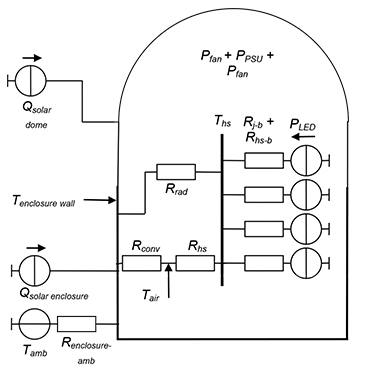Figure 2: Thermal resistance diagram of the lighting system given in its electrical equivalent.

Dimensions

The LEDs are mounted on a 200 mm x 200 mm metal core board (MCB). The heatsink base dimensions have the same area as the MCB. The heatsink is 100 mm high and is cooled by two fans dissipating 3 W together. The heatsink/fan combination has a thermal resistance of 0.6 K/W.

The enclosure is round with a diameter of 400 mm and is 400 mm high.

It is assumed that the wall thickness of the enclosure and dome has a negligible effect on the analysis. Therefore, the conduction resistance of the walls is ignored.

It is also assumed that only the enclosure transfers heat to the outside.

The dome consists of a 200 mm high straight section with the same diameter as the enclosure.

Ambient Conditions

The solar load (direct, indirect and diffuse) is assumed to apply to half the outside area of the enclosure and dome.

For the lifetime condition, the solar load is 700 W/m² at an ambient of 20°C.

For the maximum condition, the solar load is 1020 W/m² at an ambient of 40°C.

The solar absorptivity, αsolar-dome, of the dome is assumed to be 0.4.

The solar absorptivity, αsolar-EN, of the enclosure is assumed to be 0.4.

The emissivity of the enclosure is assumed to be 0.85.

The calculations will be done at no wind and with a wind speed of 2 m/s using the heat transfer coefficients of 5 and 15 W/m²·K, respectively.

LEDs
A total of 25 cold white LEDs will be evaluated at 350 to 1000 mA. The evaluation uses the LUXEON Rebel reliability and lifetime data for the B10, L70 condition with required lifetime of 40,000 hours. The lifetime condition B10, L70 implies that for a specific lifetime, 10% of the LEDs are expected to fail at the specified junction temperature and forward current. The failure criterion is when the light output of the LED has been reduced to 70% of its original light out.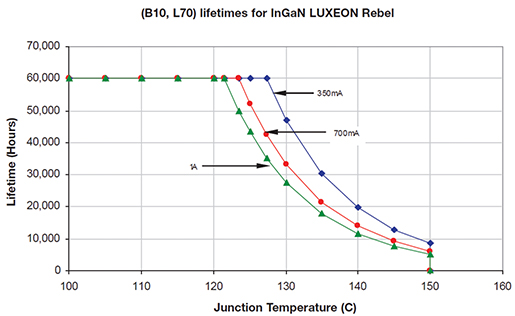Figure 3: Expected (B10, L70) lifetimes for Ingan LUXEON Rebel LEDs.1

The required junction temperatures are calculated using lifetime and maximum temperature conditions. The lifetime conditions are taken from Figure 3, while the maximum condition is from Reference.2 The lifetime and maximum temperatures are calculated using Equation 1 and are shown in Table 1. Although Table 1 shows that the lifetime conditions are more severe than the maximum conditions for certain forward current ratings, both lifetime and maximum conditions are evaluated in this article.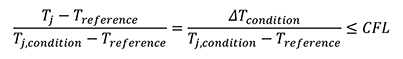(1)

 Forward Current, If [mA] Tj,lifetime condition Tamb Tj,lifetime ΔTj,lifetime 350 132.0 20.0 115.2 95.2 700 128.3 20.0 112.0 92.0 1000 126.0 20.0 110.1 90.1 Forward Current, If [mA] Tj,max condition Tamb Tj,max ΔTj,max 350 150.0 40.0 133.5 93.5 700 150.0 40.0 133.5 93.5 1000 150.0 40.0 133.5 93.5

Table 1: The maximum and lifetime junction temperatures required.1,2

The LEDs can be mounted on an FR4 PCB or a metal core board. The LEDs have a junction-to-board thermal resistance of 10 K/W. The heat dissipation of the LEDs is calculated using Equation 2, where If is the forward current in amps, Vf is the voltage in volts and ηL is the light efficiency. The values used in the study are given in Table 2.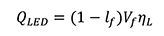(2)

 Forward current [mA] Estimated forward voltage [V] Heat dissipation [W] 350 3.2 0.896 700 3.4 1.876 1000 3.5 2.8

Table 2: LED heat dissipation assuming a 20% light efficiency.

Motor and Drivers

The motors and drivers are used in the enclosure to rotate the lighting system. The total power dissipation from these devices is assumed to be 10 W.

LED Power Supply Unit (PSU)

The LED power supply unit will also dissipate heat and is given by Equation 3, where N is the number of LEDs and ηPSU is the PSU efficiency, which is assumed to be 85%.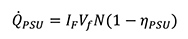(3)

Calculation procedure

The first calculation step for this analysis is to determine the outside wall temperature. This can be done by applying a control volume around the enclosure. Applying a steady-state energy balance, Equation 4, to the control volume yields Equation 5, which is solved iteratively for the enclosure wall temperature, TEN. Please note that in the calculations, the temperature is in Kelvin and not degree Celsius. This is because the radiation equations use temperature in Kelvin to calculate heat flux.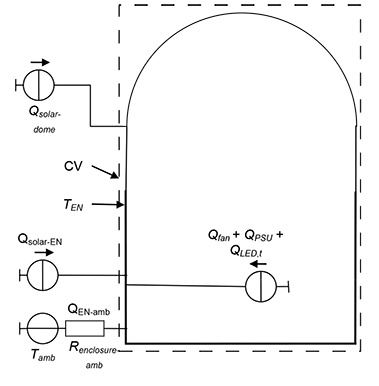Figure 4: Control volume around the enclosure wall.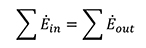(4)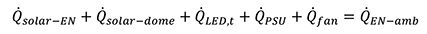(5)

Where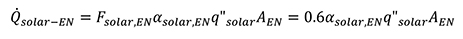(6)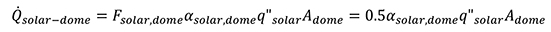(7)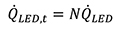(8)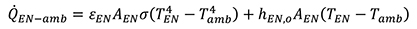(9)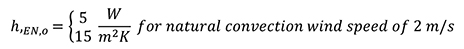(10)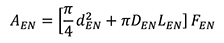(11)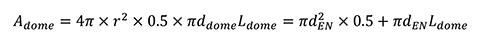(12)

The factor FEN in Equation 11 is the increase in the enclosure surface area. Any increase in this factor over 1 means that the enclosure surface has become wavy or corrugated. Therefore, it has a higher surface area than before.

The second step is to calculate the heatsink temperature. This is done by applying a control volume to the heatsink, as shown in Figure 5. Applying an energy balance equation to the control volume yields Equation 13 which is then solved for the heatsink temperature, THS. The inside air is assumed to be well mixed. Therefore, the heat transfer coefficient in Equation 14 is assumed to be 8 W/m²·K.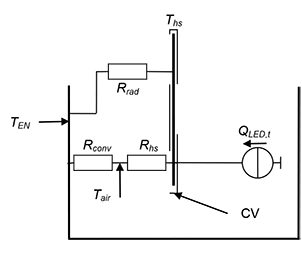Figure 5: Control volume around heatsink.(13)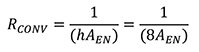(14)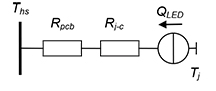Figure 6: Resistance diagram from heatsink to LED junction.

The junction temperature can be calculated applying conduction resistance from the LED junction to the heatsink, as shown in Equation 15. This can then be rearranged to Equation 16 to calculate the LED junction temperature.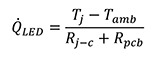(15)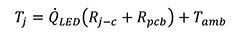(16)

Several studies have been performed to analyze the various parameters of the lighting system. A summary of these studies is listed in Table 3.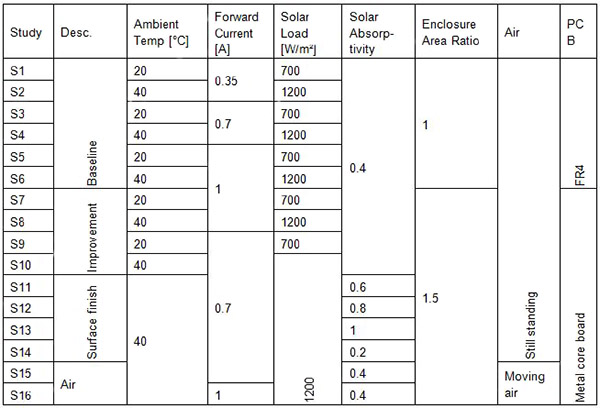Table 3: Summary of the studies to be performed.

Results

Calculation results are given in Table 4 for different case studies. Figure 7(a) shows the temperature difference between components. This gives a visual indication of the largest temperature differences and where improvements can be made. Figure 7(c) shows the heat energy applied to the system from various sources as an absolute value. Values in Figure 7(d) are similar to (c), but are given as a percentage of the total.

For the baseline model, the studies show that LED junction temperatures are above specification when the LEDs are run at 700 mA (S3 and S4) and 1000 mA (S5 and S6). When the improved model was analyzed, it was found that the junction temperature was still above specification for 1000 mA. However, for 700 mA, it was found that the junction temperatures were within specification. When the other temperatures were examined, it was found that the air temperature was 99°C. This will be far too high for the fans, PSU, motor and drivers. Considering the maximum LED storage temperature of 135ºC,2 it can be concluded that the components of the LED, e.g. encapsulate, are at too high a temperature.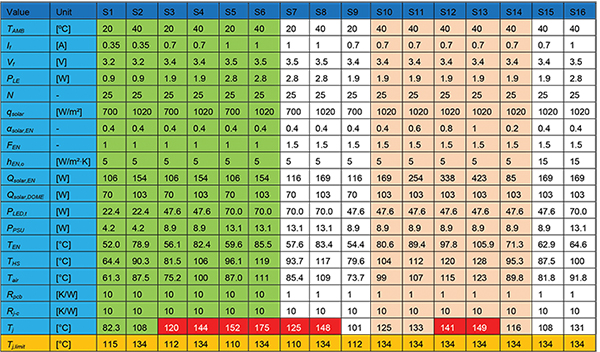Table 4: Summary of results.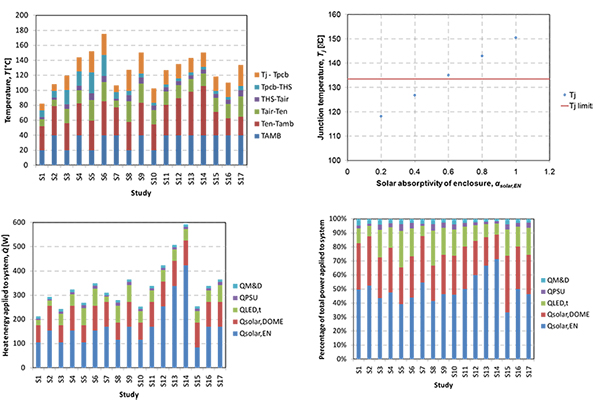Figure 7: Temperature difference between system components (a), junction temperatures as a function of solar absorptivity (b) and heat energy applied to system shown in absolute values (c) and percentage of total (d).

Though the previous studies have shown that 700 mA LEDs are not feasible for the baseline model, the improved model was also studied for the effect of change in the solar absorptivity of the enclosure wall. Although the heat load to the system has been reduced by 272 W (S11) to 188 W (S15), the air temperature remains too warm. Also, they are considered to be too shiny for a surface to have an absorptivity of 0.2. Over time, the shininess will be reduced by a dust layer, wind born dust that sand papers the surface.

Looking at the temperature difference plot in Figure 7(a) for S11, the largest temperature difference is between the enclosure and ambient. It is shown in Figure 7(d) that the solar load to the enclosure is 50% of the total heat load to the system. Because the enclosure solar load is 50% of the total load, it is recommended that a solar shield be used to decrease the temperature difference between the enclosure and ambient. The drawback of a solar shield is that wind will have a smaller effect on the system when no solar shield is present. In S16, it was shown that the junction temperature decreases by 17°C when the system is in a wind of 2 m/s.

The second largest temperature difference is between the enclosure and internal air. To decrease this temperature difference, the air inside the enclosure must be able to move along all surfaces of the enclosure and dome. This will increase the heat transfer coefficients and can be done by baffling the inside of the enclosure. However, this must be analyzed by computational fluid dynamics.

For the baseline study, it was shown that at 350 mA (S1), the junction temperature is within specification for the lifetime condition. However, the air temperature is quite high for the maximum condition (S2). This was improved by using the superior model (S7).

Summary

This article has shown how to set up an analytical model of an outside system and perform severity studies. It was found that the solar load for the enclosure was on average 50% of the entire heat load for the system. This shows that for an outside system, the solar radiation and therefore the solar absorptivity are important parameters to consider when designing the system. The system is not feasible for a forward current of 1000 mA, while further improvements would have to be evaluated using computational fluid dynamics to determine whether 700 mA is feasible. When the LEDs were run at 350 mA with the improved model, they were within specification. The air temperature was also found to be reasonable for the fans, power supply unit, motor and drivers.

Safety is also an important factor in a system design. One of the parameters needed for CE certification is that the surface temperature not be above 75°C. None of the maximum condition studies with still air were found to satisfy this condition. However, when the effect of wind was included, it was found the enclosure temperature is reduced.

References:

1. LUXEON, LUXEON® Rebel Reliability Datasheet RD07, 2007.
2. LUXEON, LUXEON® Rebel Technical Datasheet DS56, 2009.

Disclaimer: The opinions, beliefs, and viewpoints expressed by the various authors and/or forum participants on this website do not necessarily reflect the opinions, beliefs, and viewpoints of Digi-Key Electronics or official policies of Digi-Key Electronics.